# Types of Price Elasticity of Demand

## Notes

### Types of Price Elasticity of Demand :

1) Perfectly Elastic Demand (Ed = ∞) :
When a slight or zero change in the price brings about an infinite change in the quantity demanded of that commodity, it is called perfectly elastic demand. It is only a theoretical concept. For example, 10% fall in price may lead to an infinite rise in demand.

"Ed"="Percentage change in Quantity Demanded"/"Percentage change in Price"="∞"

Ed = ∞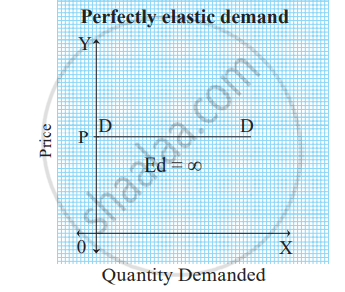In the figure above, the demand curve DD is a horizontal line parallel to the X axis indicating perfectly elastic demand.

2) Perfectly inelastic demand (Ed = 0) :
When a percentage change in price has no effect on the quantity demanded of a commodity it is called perfectly inelastic demand. For example, 20% fall in price will have no effect on quantity demanded.

"Ed"="%ΔQ"/"%ΔP"

"Ed"="0"/"20"="0"

"Ed"="0"

In practice, such a situation rarely occurs. For example, demand for salt, milk.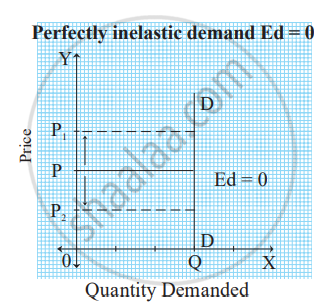In the figure above, when price rises from OP to OP1 or when price falls from OP to OP2, demand remains unchanged at OQ. Therefore, the demand curve is a vertical straight line parallel to the Y axis, indicating perfectly inelastic demand.

3) Unitary elastic demand (Ed = 1) :
When a percentage change in price leads to a proportionate change in quantity demanded then demand is said to be unitary elastic. For example, 50% fall in price of a commodity leads to 50% rise in quantity demanded.

"Ed"="%ΔQ"/"%ΔP"="50"/"50"="1"

∴ Ed = 1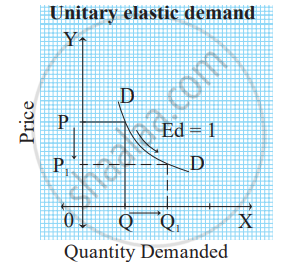In the figure above, when price falls from OP to OP1 (50%), demand rises from OQ to OQ1 (50%). Therefore, the slope of the demand curve is a 'rectangular hyperbola'.

4) Relatively elastic demand (Ed >1) :
When a percentage change in price leads to more than proportionate change in quantity demanded, the demand is said to be relatively elastic. For example, 50% fall in price leads to 100% rise in quantity demanded.

"Ed"="%ΔQ"/"%ΔP"

"Ed"="100"/"50"

∴ Ed = 2

Ed > 1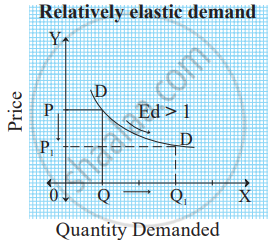In the figure above, when price falls from OP to OP1 (50%), demand rises from OQ to OQ1 (100%). Therefore, the demand curve has a flatter slope.

5) Relatively inelastic demand (Ed < 1) :
When a percentage change in price leads to less than proportionate change in the quantity demanded, demand is said to be relatively inelastic. For example, 50% fall in price leads to 25% rise in quantity demanded.

"Ed"="%ΔQ"/"%ΔP"="25"/"50"="0.5"

"Ed"="0.5"

∴ Ed < 1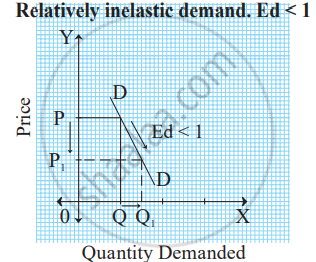In the figure above, when price falls from OP to OP1 (50%), demand rises from OQ to OQ1 (25%). Therefore, the demand curve has a steeper slope.

#### Description Percentage

Ed = 0 Perfectly inelastic Change in price does not affect demand
at all.
Ed = ∞ Perfectly Elastic Slight change in price brings infinite
change in demand.
Ed = 1 Unitary Elastic Change in demand is equal to change in
price.
Ed > 1 Relatively Elastic Change in demand is more than change in price.
Ed < 1 Relatively Inelastic Change in demand is less than change in price
If you would like to contribute notes or other learning material, please submit them using the button below.